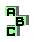NINECENTER   (3/6)

 r1179 altitude feet, incenter, circumcenter r1180 altitude feet, incenter, circumcenter, orthologic triangles r1182 altitude feet, circumcenter r1183 altitude feet, circumcenter, centroid, cevian quotient r1219 centroid, orthocenter, Euler line, homothetic triangles r1231 concyclic points r1268 altitude feet, pedal circle r1269 altitude feet, orthocenter r1308 complement r1379 Steiner deltoid, ninecircle, homothetic triangles r1382 Steiner deltoid, circumcircle r1387 Steiner deltoid, ninecircle, homothetic triangles r1402 Jerabek hyperbola, point X(186), point X(265) r1421 circumcevian triangle, orthocenter, circumcenter r1455 symmedian point, Prasolov point, point X(155) r1486 r1487 orthologic triangles, circumcenter, antigonal points r1520 radical center r1534 orthocenter, circumcenter r1547 Vecten points, cevian product, symmedian point, altitudes r1554 circumcenter, circumcevian triangle r1592 incenter, Feuerbach point, point X(65) r1593 incenter, Feuerbach point r1603 circumcenter, Euler line r1611 orthocenter, circumcenter, altitude feet, isogonal points1 2 3 4 5 6